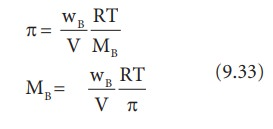Home | | Chemistry 11th std | Determination of molar mass from osmotic pressure

# Determination of molar mass from osmotic pressure

According to vanâ€™t Hoff equation Ï€=cRT, c= n / V

Determination of molar mass from osmotic pressure

According to vanâ€™t Hoff equation

Ï€=cRT

c= n / V

Here, n= number of moles of solute dissolved in â€˜Vâ€™ litre of the solution.

Therefore, Ï€ = n/V . RT or

Ï€V=nRT (9.32)

If the solution is prepared by dissolving wB g of nonvolatile solute in wA g of solvent, then the number of moles â€˜nâ€™ is,

n= wB/MB

Since,MB = molar mass of the solute

Substituting the â€˜nâ€™ in 9.32, we get,From the equation 9.33, molar mass of the solute can be calculated.

Study Material, Lecturing Notes, Assignment, Reference, Wiki description explanation, brief detail
11th Chemistry : UNIT 9 : Solutions : Determination of molar mass from osmotic pressure |